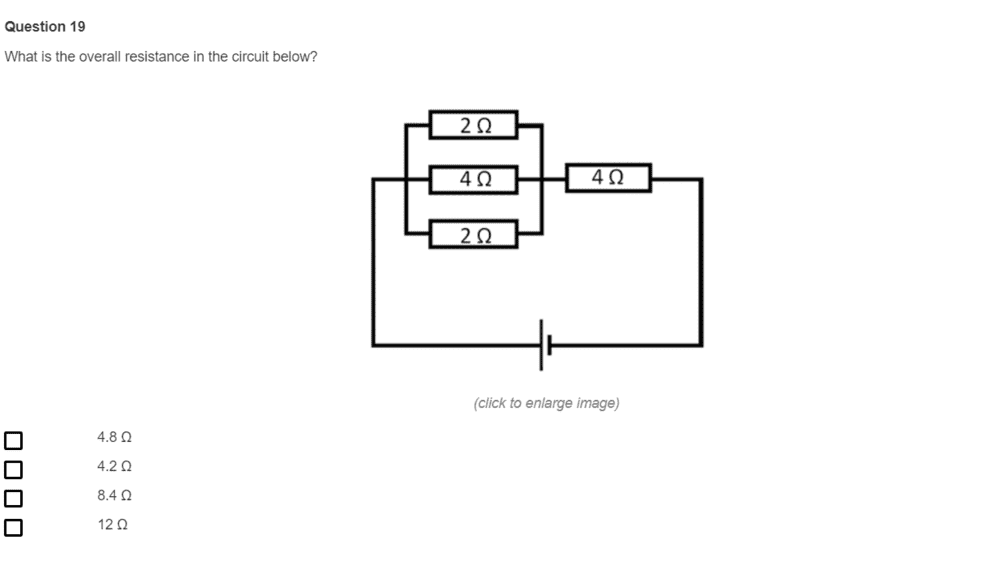# How to calculate Resistors in a circuit ?

Homework Statement:
i tried to solve for this question as far as i know to calculate the resistors you only need to add all of them but my answer of 12 was wrong
Relevant Equations:
r1+r2+r3.....
here is the question below with all the possible answers, if anyone can help it would be appreciatedOrodruin
Staff Emeritus
Homework Helper
Gold Member
You only add resistors that are connected in series. What do you do with resistors that are connected in parallel?

•JD_PM
You only add resistors that are connected in series. What do you do with resistors that are connected in parallel?
for parallel i use the formula 1/r1+1/r2+1/r3
but this one is a complex of both parallel and a series

Orodruin
Staff Emeritus
Homework Helper
Gold Member
Start by replacing the parallel resistors by an equivalent single resistor.

Start by replacing the parallel resistors by an equivalent single resistor.
1/4 + 1/4 = 2 that's the total of the parallel resistors but from there i don't know how to add it to the sum of the series resistors to get the total answer

Orodruin
Staff Emeritus
Homework Helper
Gold Member
1/4 + 1/4 = 2 that's the total of the parallel resistors
This is not correct. There are three parallel resistors, not two.

Edit: Also, it should obviously be 1/(1/4 + 1/4) = 2.

This is not correct. There are three parallel resistors, not two.

Edit: Also, it should obviously be 1/(1/4 + 1/4) = 2.
its the same answer (2) as mine
but the problem is i don't know how to add it to the other ohms in series so i can get the total resistance

Orodruin
Staff Emeritus
Homework Helper
Gold Member
its the same answer (2) as mine
My point is that you quoted the computation wrong. Also, the answer is wrong because there are three resistors in parallel, not two. Also, there are not two 4 Ω resistors that are connected in parallel.

So let us go back to the basics: Which resistors in your figure are connected in parallel? Which resistors are connected in series?

4 Ω and the other 4 Ω are in parallel while 2 Ω and 2 Ω are in series

Orodruin
Staff Emeritus
Homework Helper
Gold Member
4 Ω and the other 4 Ω are in parallel while 2 Ω and 2 Ω are in series
No. This is incorrect. Look up the definition of series and parallel.

No. This is incorrect. Look up the definition of series and parallel.
so 2 Ω and 2 Ω and 4 Ω are in parallel and the 4 Ω alone is in series
i have to sum the parallel ohms to replace them with a single resistor of 8 right ?

Orodruin
Staff Emeritus
Homework Helper
Gold Member
so 2 Ω and 2 Ω and 4 Ω are in parallel and the 4 Ω alone is in series
i have to sum the parallel ohms to replace them with a single resistor of 8 right ?
No. It is correct that the 2-2-4 combination is in parallel. However, you did not use the correct relation to get the equivalent resistance to replace that entire combination.

No. It is correct that the 2-2-4 combination is in parallel. However, you did not use the correct relation to get the equivalent resistance to replace that entire combination.
inverse 1/2 + 1/2 + 1/4 = 0.8
then i add 0.8 to the single 4 Ω and the sum = 4.8

i was only confused by the parallel and series situation as the circuit is rotated
thank you

Last edited: